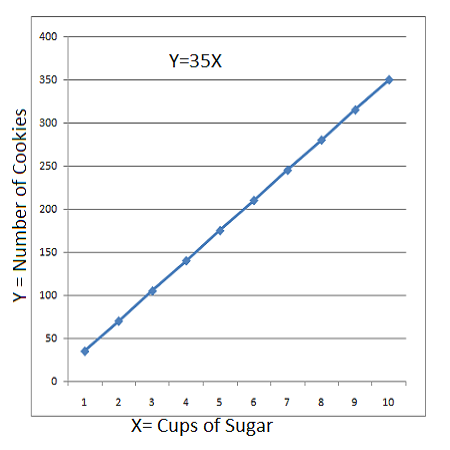# A cookie company uses one cup of sugar for every 35 cookies it makes. Let x represent the total...

## Question:

A cookie company uses one cup of sugar for every 35 cookies it makes. Let x represent the total number of cups of sugar used, and let y represent the number of cookies made. Write an equation relating to x, and y then graph your equation.

## Algebraic Equation Word Problems:

An algebraic equation is formed when a relation between any variable is equated to a constant. In word problems involving an algebraic equation, this relation is given in the problem statement and we need to form an algebraic equation out of it. Assuming a base variable and determining other unknowns in its term also helps in simplifying the equation.

For example: {eq}4x +3y = 1{/eq} is an algebraic equation where {eq}x{/eq} and {eq}y{/eq} are variables and {eq}1{/eq} is the equivalent constant of the relation shown in the equation.

Let {eq}x{/eq} represent the total number of cups of sugar used, and let {eq}y{/eq} represent the number of cookies made

It was given that {eq}1{/eq} cup of sugar is used to make {eq}35{/eq} cookies.

Then, {eq}x{/eq} cup of sugar is used to make {eq}35x{/eq} cookies.

Thus, it can be expressed as:

\begin{align} \rm Number\ of\ cookies\ made\ &=\rm Cookies\ made\ with\ 1\ cup\ sugar \times Number\ of\ cups \\[0.2cm] y &= 35x \end{align}